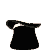I'll guess what number you're hiding.

Choose any number, for example 7146.
Cross one of the digits out (except for 0), 4 for example.
Then you get a number with one digit less.
Subtract the sum of the digits of the number you first chose ( 7+1+4+6 = 18).
Give me your result (you got 698).

# So I'm going to find out the number you hid !Result of your difference  : You crossed out number :